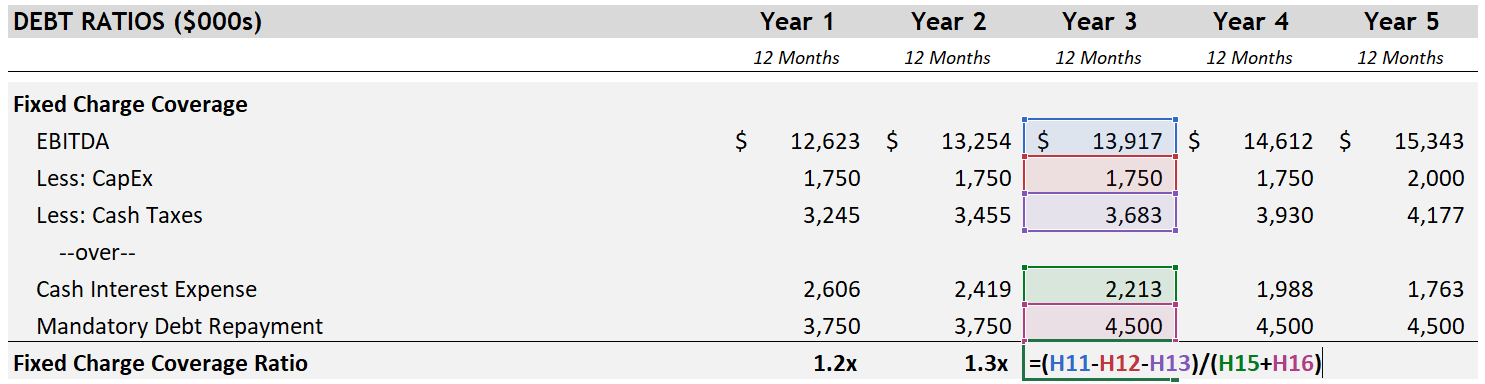Select Page

# Fixed Charge Coverage Ratio (FCCR) in Private Equity Transactions

• Peter Lynch

The fixed charge coverage ratio is used to measure a company’s ability to cover its “fixed charges” (largely debt-related payments but this can include additional obligations as you will see below) due in any given period. The definition provided here and elsewhere generally refers to “fixed charges,” which can be a little frustrating (akin to a dictionary defining “legendary” as “based on legends”). To clarify, we will start with a simple visual and expand on this by including the definition a senior lender might use in a term sheet.Formula from the image above:

( EBITDA – Capital Expenditures – Cash Taxes ) / ( Cash Interest Expense + Scheduled Debt Amortization )

The Fixed Charge Coverage Ratio gets more precise than the debt-to-EBITDA ratio by subtracting additional uses of cash from EBITDA to get to a closer approximation of cash flow for the period. The logic behind subtracting capital expenditures instead of depreciation and amortization (the “DA” in EBITDA) is that capital expenditures are a cash outflow whereas D&A are noncash items. After that cash taxes are subtracted to arrive at a better approximation of cash flow. Interest expense is not subtracted, but it can be found in the denominator (interest expense is one of the “fixed charges”).

To elaborate on the denominator, this calculation looks at the actual cash required to remain in compliance in each period. Since you are more closely comparing the cash available for debt payments to the debt payments required in each period, a lender typically requires that this ratio remain above a minimum threshold of 1.2x.

Example of what you might find in a senior debt term sheet:

“Fixed Charge Coverage Ratio – Borrower shall not permit the ratio of (a) EBITDA minus the sum of (i) capital expenditures (excluding financed or equity funded capital expenditures), (ii) taxes, (iii) distributions, divided by (b) the sum of (i) cash interest expense and (ii) scheduled principal payments on total funded debt (including capital lease payments) to be less than 1.25x.”

Notes on FCCR:
1. It is not uncommon to see capital expenditures described as “unfunded capital expenditures” in the credit agreement. If capital expenditures are “funded” it suggests that additional debt was used to purchase the equipment. The debt raised offsets the cash outflow, and the interest and principal payments associated with this new debt end up in the denominator.
2. Mandatory Debt Repayment might also be referred to as Scheduled Debt Amortization. The purpose is to include only the scheduled principal payments described in the credit agreement. Any optional repayment of debt would be excluded as would any repayment of debt under a cash flow sweep.
3. Finally, lease payments might also be included in the denominator with cash interest expense and debt repayment.